# Solve the given initial-value problem. x=(-1 12}x.xco= (-4) X(t) = 12e9t - 13(3t-1)9, 4e9t + 13te...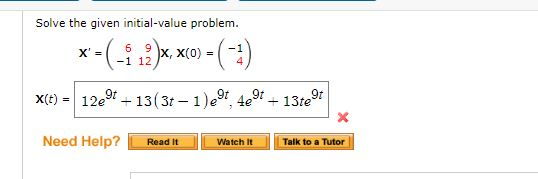Solve the given initial-value problem. x=(-1 12}x.xco= (-4) X(t) = 12e9t - 13(3t-1)9, 4e9t + 13te Need Help? Read It Watch it Talk to a Tutor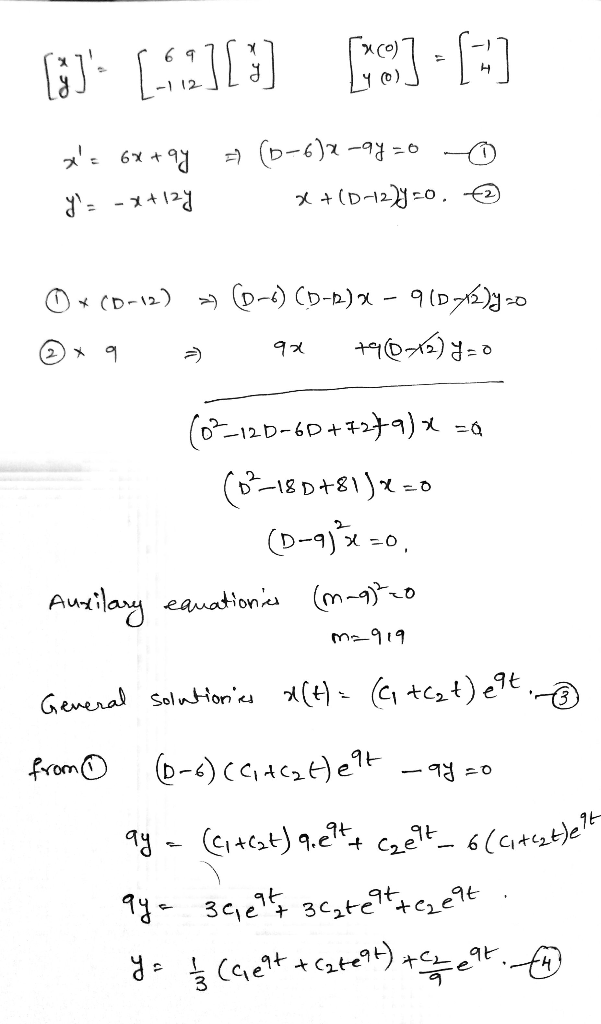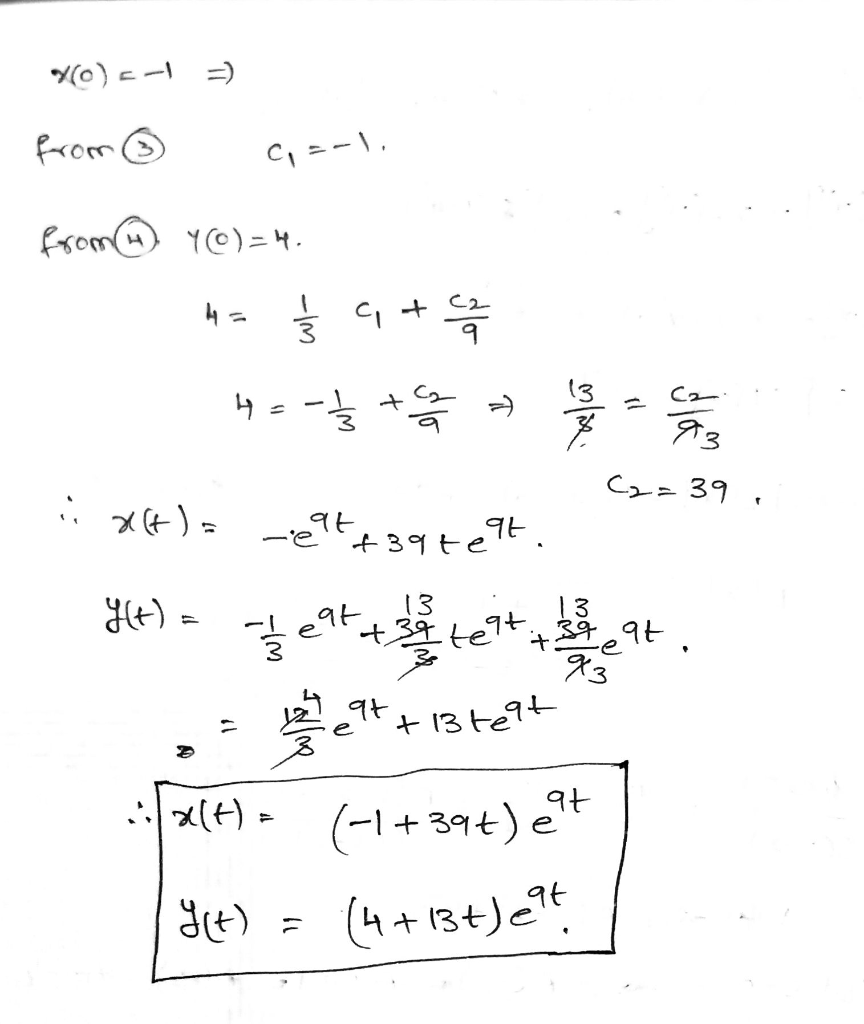##### Add Answer of: Solve the given initial-value problem. x=(-1 12}x.xco= (-4) X(t) = 12e9t - 13(3t-1)9, 4e9t + 13te...
Similar Homework Help Questions
• ### Solve the given initial-value problem. -1 -2 JX + 4 X(t) Need Help? Read It Watch...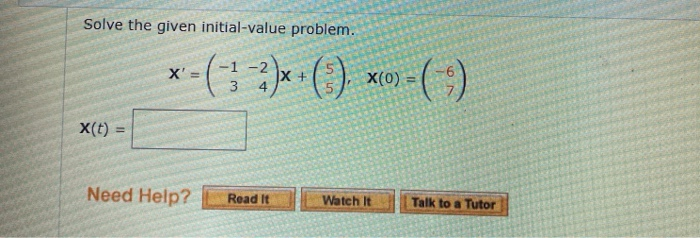Solve the given initial-value problem. -1 -2 JX + 4 X(t) Need Help? Read It Watch It Talk to a Tutor

• ### 9. -/1 points ZILLDIFFEQMODAP11 2.5.029. My Note Solve the given initial-value problem. The DE is of...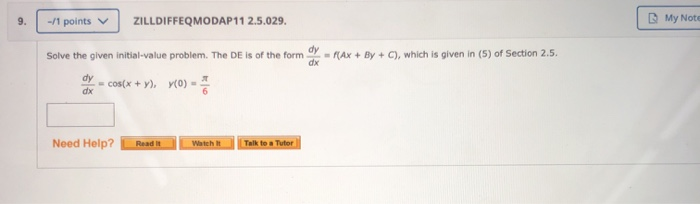9. -/1 points ZILLDIFFEQMODAP11 2.5.029. My Note Solve the given initial-value problem. The DE is of the form - FAX + By + C), which is given in (5) of Section 2.5. x = cos(x + ). YO) Need Help? Read It Watch It Talk to a Tutor

• ### 9. [0/1 Points] DETAILS PREVIOUS ANSWERS ZILLDIFFEQMODAP11 5.1.0. Solve the given initial-value problem. d2x dt2 +...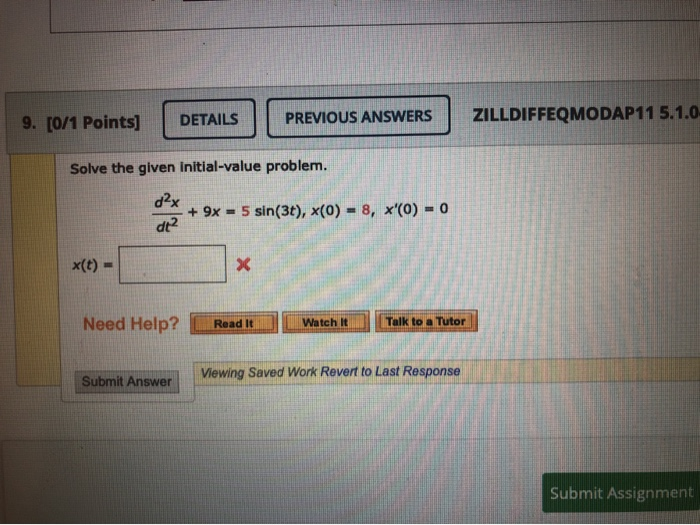9. [0/1 Points] DETAILS PREVIOUS ANSWERS ZILLDIFFEQMODAP11 5.1.0. Solve the given initial-value problem. d2x dt2 + 9x = 5 sin(3), (0) = 8, x'(0) = 0 x(t) = X Need Help? Read It Watch it Talk to a Tutor Submit Answer Viewing Saved Work Revert to Last Response Submit Assignment

• ### Solve the given initial-value problem by finding, as in Example 4 of Section 2.4, an appropriate...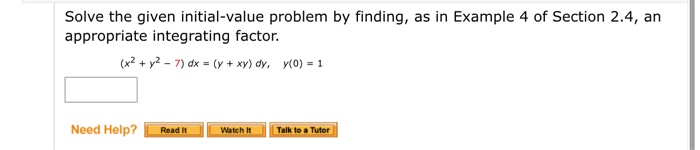Solve the given initial-value problem by finding, as in Example 4 of Section 2.4, an appropriate integrating factor. (x2 + y2 - 7) dx = (y + xy) dy, y(0) = 1 Need Help? Read It Watch It Talk to a Tutor

• ### Solve the given initial-value problem. dax + 4x = -7 sin(2t) + 6 cos(2t), x(0) =...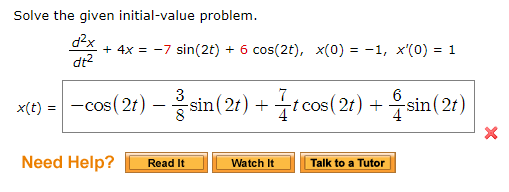Solve the given initial-value problem. dax + 4x = -7 sin(2t) + 6 cos(2t), x(0) = -1, x'(0) = 1 xce) = -cos(2+) – sin(2t) + {cos(21) + (sin(21) Need Help? Read It Watch It Talk to a Tutor

• ### 2. 0/10 points | Previous Answers ZillDiffEQ9 8.2.048 Solve the given initial-value problem 6-1)X, X'=( X(0)= Need Help? Read It Talk to a Tutor 2. 0/10 points | Previous Answers ZillDiffEQ9...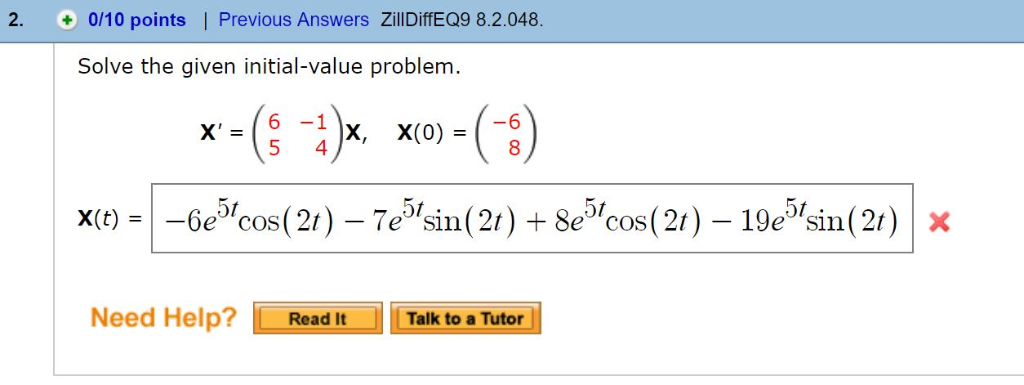2. 0/10 points | Previous Answers ZillDiffEQ9 8.2.048 Solve the given initial-value problem 6-1)X, X'=( X(0)= Need Help? Read It Talk to a Tutor 2. 0/10 points | Previous Answers ZillDiffEQ9 8.2.048 Solve the given initial-value problem 6-1)X, X'=( X(0)= Need Help? Read It Talk to a Tutor

• ### 18. + 14 points ZillDiffEQ9 8.2.031. Solve the given initial-value problem. 6 9 )X, X(0)=( x1...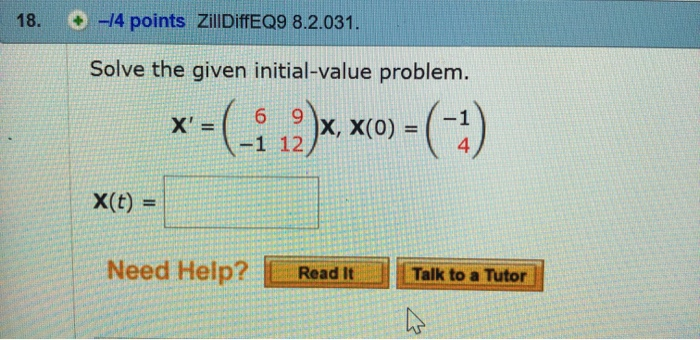18. + 14 points ZillDiffEQ9 8.2.031. Solve the given initial-value problem. 6 9 )X, X(0)=( x1 12/ 4 x(t) = Need Help? ReadTalk to a Tutor

• ### Use the Laplace transform and the procedure outlined in Example 10 to solve the given boundary-value problem. y(t) =| e-t + 6(1 + t)-e-t(1-6) | X Need Help? Talk to a Tutor Read it Use the Lapla...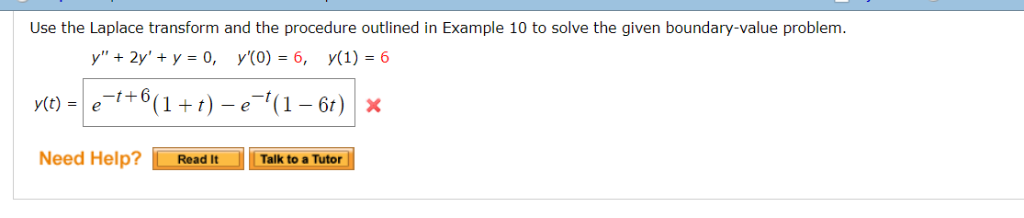Use the Laplace transform and the procedure outlined in Example 10 to solve the given boundary-value problem. y(t) =| e-t + 6(1 + t)-e-t(1-6) | X Need Help? Talk to a Tutor Read it Use the Laplace transform and the procedure outlined in Example 10 to solve the given boundary-value problem. y(t) =| e-t + 6(1 + t)-e-t(1-6) | X Need Help? Talk to a Tutor Read it

• ### Use variation of parameters to solve the given nonhomogeneous system. X' = ( X + -1...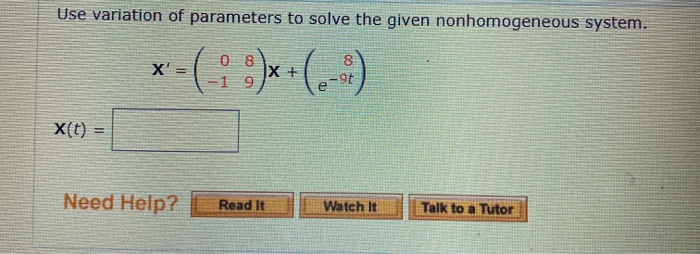Use variation of parameters to solve the given nonhomogeneous system. X' = ( X + -1 9 9t e X(t) = Need Help? Read It Watch It Talk to a Tutor

• ### -14 POINTS ZILLDIFFEQMODAP11 7.2.041. Use the Laplace transform to solve the given initial-value pro y"' +...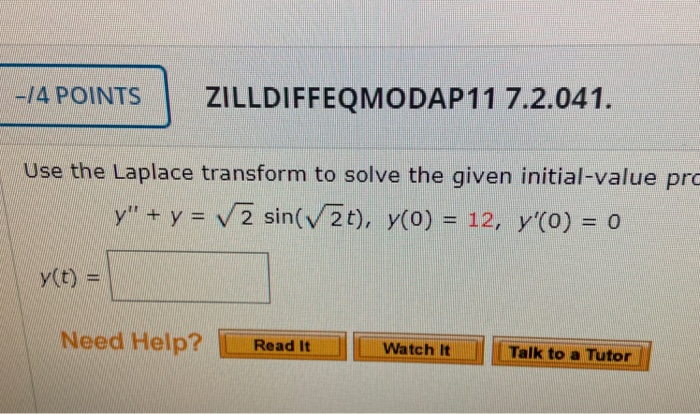-14 POINTS ZILLDIFFEQMODAP11 7.2.041. Use the Laplace transform to solve the given initial-value pro y"' + y = sin(V2t), y(0) = 12, y'(O) = 0 y(t) = Need Help? Read It Watch It Talk to a Tutor

Free Homework App